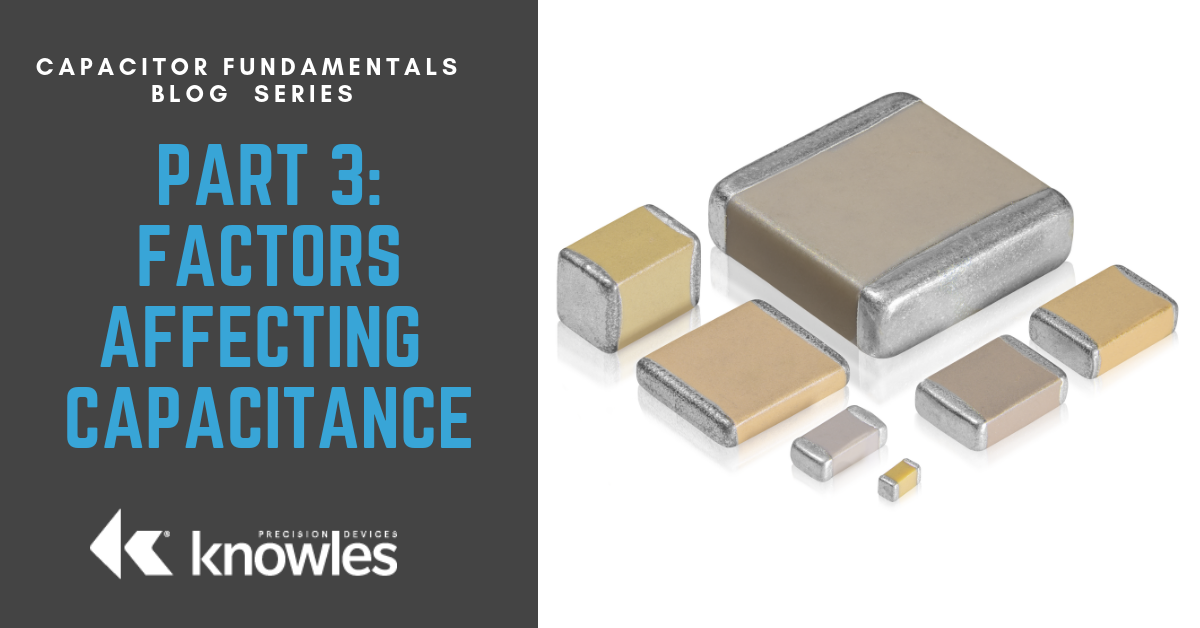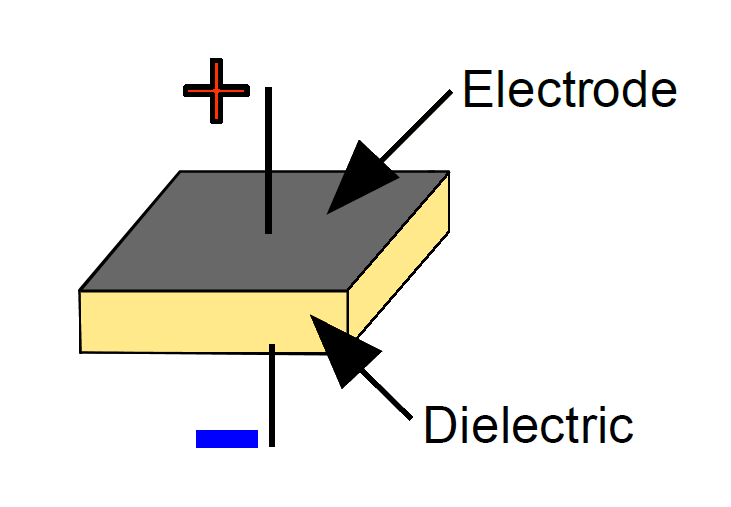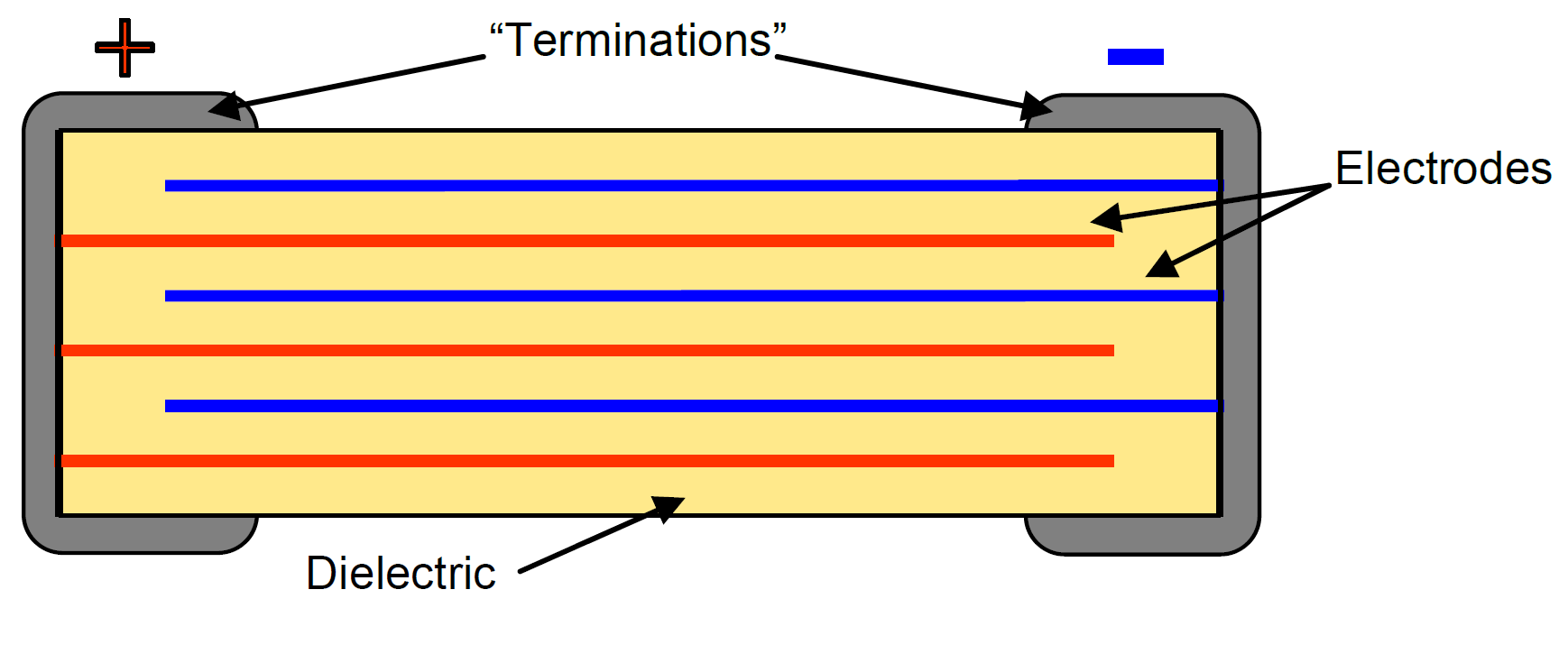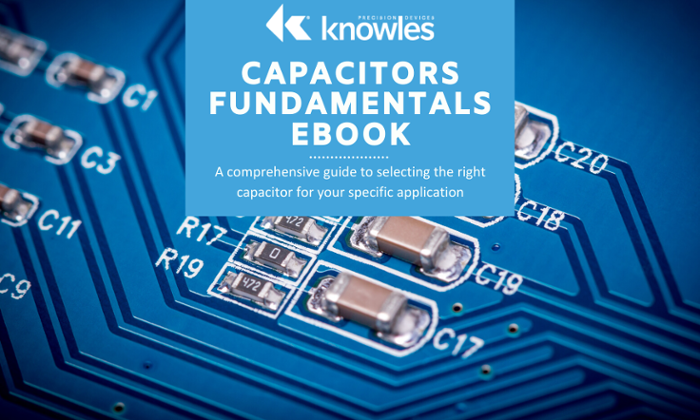Welcome to the Capacitor Fundamentals Series, where we teach you about the ins and outs of chips capacitors – their properties, product classifications, test standards, and use cases – in order to help you make informed decisions about the right capacitors for your specific applications. After describing common applications for capacitors in our previous article, let’s consider the factors and limitations that affect capacitance.For any given voltage, the capacitance value of the single plate device (see Figure 1) can be calculated from the geometry and dielectric constant of the device as follows:

C = KA/f(t)

whereC = capacitance

K = dielectric constant

A = area of electrode

t = thickness of dielectric

f = conversion factor

Figure 1. Single Layer Capacitor

In the English system of units, f = 4.452, the dimensions for A and t are listed in inches, and the capacitance value is expressed in picofarads (pF). Therefore, for a device such as in Figure 1 with a 1.0” X 1.0" area, 0.056" dielectric thickness, and a dielectric constant of 2500, the capacitance comes out to:

C = 2500 (1.0)(1.0)/4.452 (0.056) = 10,027 pF

Utilizing the metric system, the conversion factor is f = 11.31 and dimensions are used in centimeters. The capacitance comes out to the same value of:

C = 2500 (2.54)(2.54)/11.31 (0.1422) = 10,028 pF

As seen in the above equation, greater capacitance can be achieved by increasing the electrode area while decreasing the dielectric thickness. Since increasing the area in a single plate device with thinner dielectric will produce a fragile, unusable component, the concept of stacking capacitors in a parallel array was conceived to produce a physically sound device with more capacitance per unit volume, as illustrated in Figure 2.Figure 2. Multilayer capacitor

In this multilayer configuration, the area A is increased by virtue of many electrodes in parallel arrangement, in a construction permitting very thin dielectric thickness between opposing electrodes. Therefore, the capacitance C is enlarged by the factor N (number of dielectric layers) and reduced dielectric thickness t’, where A’ is now the area of overlap of opposing electrodes:

C = KA’N/4.452(t’)

The capacitance value previously obtained for the 1.0” x 1.0” x 0.056" single plate device can now be produced with the same dielectric in a multilayer unit of only 0.050”x 0.050” x 0.040” dimensions and 30 dielectric layers of thickness 0.001" (where the electrode overlap A’ is 0.030” x 0.020").

C = 2500 (0.030) (0.020) 30/4.452 (0.001) = 10,107 pf

This example shows how multilayer construction can deliver the same capacitance in a volume 700 times smaller than that of the single plate device. Chip capacitors are therefore designed and manufactured to maximize the volumetric efficiency of capacitance by optimizing the geometry and by selecting dielectric formulations with high dielectric constant and general electrical properties (e.g., good insulation resistance and dielectric strength), which permit very thin layer construction.

As a final consideration, these relationships have knock-on effects on the amount of capacitance available at set sizes and voltages. Smaller footprint and restricted thickness limit the available capacitance value. Higher voltage capacitors need greater dielectric thickness, which has the effect of reducing the capacitance in a nonlinear fashion. For example, increasing the voltage from 1000 V to 2000 V requires a typical doubling of dielectric thickness; this, in turn, means that only half the number of electrodes can fit into a set thickness. Due to the fact that capacitance is directly proportional to overlap area and inversely proportional to dielectric thickness, the overall capacitance available in a given size at 2000 V is now roughly 25% of that at 1000 V.

Hopefully, Part 3 gave you a better understanding of the main parameters that affect capacitance and how they can impact your specific application. In Part 4, we’ll be covering key behaviors such as dielectric polarization and loss. Also, check out our Knowles Precision Devices Capacitors to view our complete product offering.Topics: Capacitor

## Insights Into Precision Passive Devices

Knowles Precision Devices is a premier global source for Capacitors, RF Filters, EMI Filters, Resonators, non-magnetic components and advanced dielectric materials. An umbrella for the brands of Compex, DLI, Johanson MFG, Novacap, Syfer and Voltronics, Knowles Precision Devices serves a variety of markets including military, aerospace/avionics, medical equipment, implantable devices, EMI and connector filtering, oil exploration, instrumentation, industrial electronics, automotive, telecoms and data networks.

This Blog will provide insights into:

• Filters for 5G and mmWave
• Capacitors for High Voltage
• Capacitors for High Reliability

and much more. Subscribe below to stay informed.